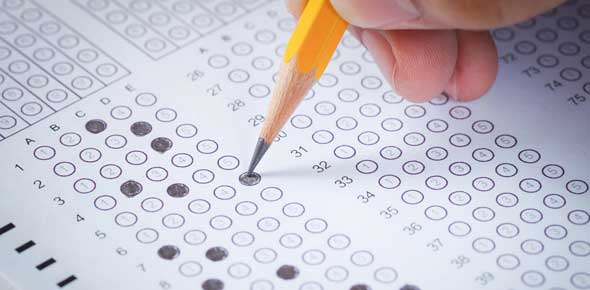# Science Quiz For TExES Test

8 Questions | Total Attempts: 118SettingsThis is a sample test to gauge your knowledge on science quiz for TexES test. Don’t be scared by this rather complex initial, it is not as hard. Take it to find out how much you know. All the best and enjoy.

• 1.
Any substance with mass that takes up space. All ______________ has mass, volume, and density.
• A.

Mass

• B.

Color

• C.

Volume

• D.

Density

• E.

Matter

• 2.
The amount of space an object takes up.
• A.

Density

• B.

Mass

• C.

Volume

• D.

Matter

• E.

Transparency

• 3.
The measure of how much mass is in a given volume.
• A.

Volume

• B.

Matter

• C.

Mass

• D.

Density

• E.

Luster

• 4.
The hue of an object; The smell of an object; How shiny a metal is;
• 5.
How well an object can be seen through; How hard an object is; How well a liquid dissolves; The ability to be flattened or bent without breaking.
• 6.
The ability to transfer heat.
• A.

Electrical conductivity

• B.

Thermal conductivity

• C.

Malleability

• D.

Disolvability

• E.

Hardness

• 7.
The ability to transfer electricity.
• A.

Electrical conductivity

• B.

Malleability

• C.

Dissolvability

• D.

Thermal conductivity

• E.

Electricity

• 8.
What are the phases of matter?
• A.

Density; volume; mass

• B.

Solid; Liquid; Gas

• C.

Kinetic Energy; Potential Energy; Stored Energy

Related Topics